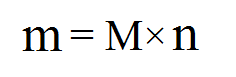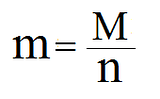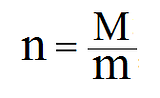Monday, September 5, 2011

Stoichiometry Note #3: Molar Mass

Previous: Converting Moles to Molecules                                          Next: Molar Ratio

What is molar mass? Well, the idea is very simple— it's basically the mass of one mole of a specific atom or molecule. For example, oxygen has a molar mass of 15.999 grams per mole, and hydrogen has a molar mass of 1.0079 grams per mole. The symbol of molar mass is a capital M.

Finding the Molar Mass of an Element:
To find the molar mass of an element, you'd have to look it up on the periodic table. In the periodic table below, the molar masses are displayed below the symbol of each element:click to enlarge

Calculating the Molar Mass of a Molecule
To calculate the molar mass of a molecule, you have to first find the molar mass of each individual element. Then, you have to multiply each molar mass by its element's subscript. After, add the values together and the molar mass of the molecule will be obtained. For example, to calculate the molar mass of water (H2O):
1. Find molar mass of each element:
• Oxygen : 15.999
• Hydrogen :1.0079
2. Multiply each molar mass by the subscript of the element (H2O1):
• Oxygen: 15.999×1= 15.999
• Hydrogen: 1.0079×2= 2.0158
3. Add the results together:
• 15.999+2.0158= 18.0148 grams/mole
Hopefully that's clear enough.

Converting Molar Mass to Mass:
To find the mass of a certain amount of molecules, use the following equationswith the variables being:
• m: mass of the molecule
• M: molar mass of the molecule
• n: the number of moles

This is quite simple. Imagine that you have three bags of tomatoes and each of them weigh 2 kilograms. To calculate the total mass, you'd multiply the mass per bag (2 kg) by the number of bags (3 bags), and you would get 6 kilograms as your answer.

Similarly, if you have three moles of a molecule, and you know that the molar mass of the molecule is 2 grams/mole, then you would multiply 2 by 3 to get 6 grams as the total mass.

You can also change the equation a bit to calculate the number of moles or the molar mass (if you have the other two variables):Worksheets:
The concept of molar mass is extremely important in stoichiometry, and make sure that you know how to do the problems in these two worksheets:

Previous: Converting Moles to Molecules                                          Next: Molar Ratio